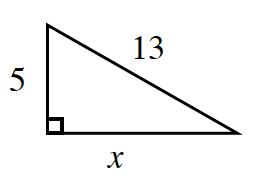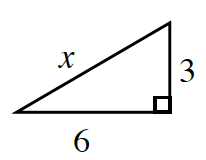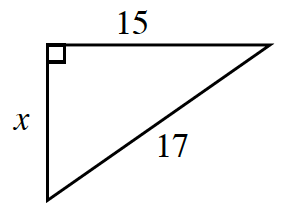### Home > CC3MN > Chapter 10 > Lesson 10.1.1 > Problem10-10

10-10.

Find the length of the side labeled $x$ in each of the right triangles pictured below. Identify each answer as a rational or irrational number.

Use the Pythagorean Theorem.

1.$(\text{leg } 1)^2 + (\text{leg } 2)^2 = (\text{hypotenuse})^2$
Substitute the values that are given.

$(5)^2 + (x)^2 = (13)^2$
$25 + x^2 = 169$

Solve by isolating $x$.

$x^2 = 144$
Take the square root of each side to get the answer.

1.$(\text{leg } 1)^2 + (\text{leg } 2)^2 = (\text{hypotenuse})^2$
Substitute the values that are given.

$(6)^2 + (3)^2 = (x)^2$
Now solve for $x$.

1.See part (a).

$8$